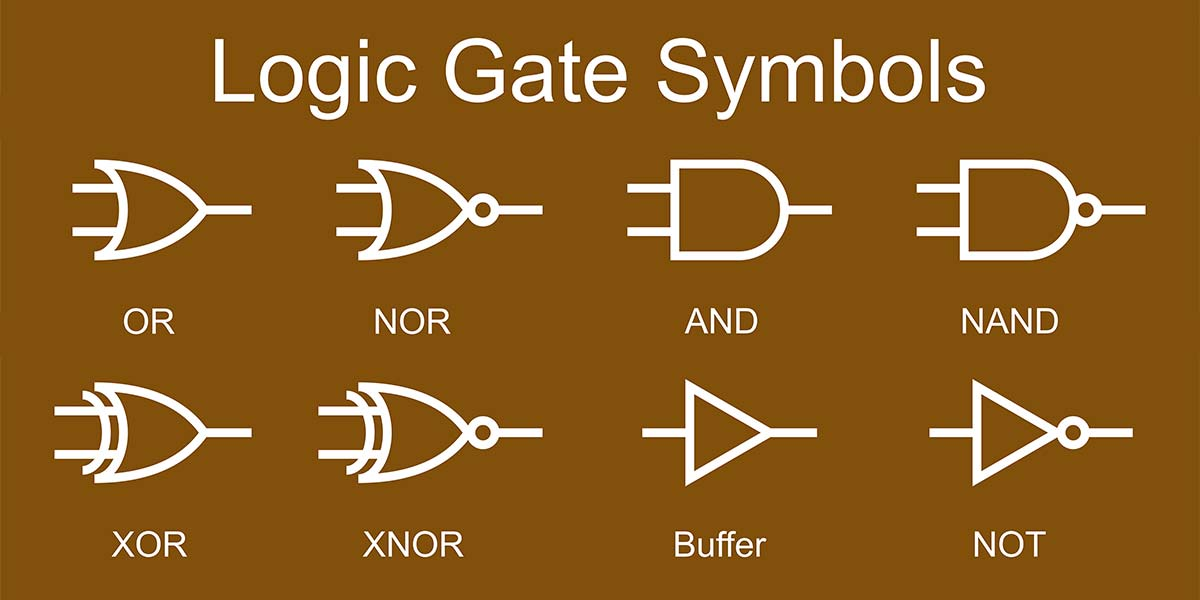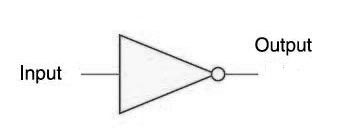# Logic Gate

Welcome to Class !!

In today’s Computer Science class, We will be learning about Logic Gates. We hope you enjoy the class!Logic Circuits

Logic gates are physical devices that perform logical operations on one or more logic inputs to produce an output. They are mainly diodes or transistors though can also be constructed using electromagnetic rays. A logic gate is an elementary building block of a digital circuit. Most logic gates have two inputs and one output. At any given moment, every terminal is in one of the two binary conditions low (0) or high (1), represented by different voltage levels. Both the input and output carry the binary valves ‘O’ which mean low or false ‘ I’  meaning high or true.not

##### Types of logic gates

###### 1. AND gate:

Suppose we have two inputs AND gate A and B, the A.B denotes A and B which are both connected to the AND gate. This gate gives a high output if all inputs are high.

 INPUT (A) INPUT (B) OUTPUT (A and B) 0 0 0 1 0 0 0 1 0 1 1 1

##### 2. NOT gate:

Also called an inverter, the Not gate implements the logical negation. The Not gate outputs a voltage representing the opposite logic level of its input i.e. when the input is 0, the output will be 1 and vice versa.

 INPUT (A) OUTPUT (B) 0 1 1 0

##### 3. OR gate:

The OR gate gives a high output If at least one of the input is high. For a 2 input circuit with input A and B then A+B denotes that A and B are both connected to the OR gate.

 INPUT (A) INPUT (B) OUTPUT (A and B) 0 0 0 1 0 1 0 1 1 1 1 1

##### Uses of standard Logic Circuit
1. Logic gates are building blocks of hardware electronic components.
2. It is used in the activation of doorbells.
3. The AND gates use to combine multiply signals
4. The NOT gate is used in building a switch.

We have come to the end of this class. We do hope you enjoyed the class?

Should you have any further question, feel free to ask in the comment section below and trust us to respond as soon as possible.

In our next class, we will be learning about Alternative Logic Gates. We are very much eager to meet you there.

Are you a Parent? Share your quick opinion and win free 2-month Premium Subscription

Don`t copy text!